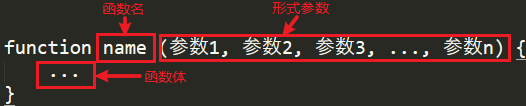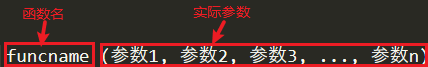# 【php基础入门】细说PHP中的函数声明与使用详解（重要）

## 一、PHP函数

$table = "<table border='1' cellspacing='0' fontSize='32' bgColor='red'>"; for($i=0;$i<5;$i++){
$table .="<tr>"; for($j=0;$j<3;$j++){
$table .= "<td>帅</td>"; }$table .="</tr>";
}
$table .= "</table>"; echo$table;


function createTable(int $rows,int$cls,string $bgColor="red",string$content="帅"){
$table = "<table border='1' cellspacing='0' fontSize='32' bgColor='{$bgColor}'>";
for($i=0;$i<$rows;$i++){
$table .="<tr>"; for($j=0;$j<$cls;$j++){$table .= "<td>{$content}</td>"; }$table .="</tr>";
}
return $table .= "</table>"; } echo createTable(3,2,'green');  系统函数（内置的提前定义好的，直接调用即可） // 绝对值函数 echo abs(-3.1415926); // 3.1415926 // 字符串长度 echo strlen('nihao'); // 5 echo strlen('你好'); // 6个字符 // 小写转大写 echo strtoupper('hello world'); // HELLO WORLD // 生成随机数 echo mt_rand(0,100); // 数组长度 echo count([1,2,3,4]); // 4  ### 1. PHP定义函数 #### 命名函数 在 PHP 中声明一个自定义的命名函数可以使用下面的语法格式： function 函数名称(类型限定 参数列表): 返回值类型 { // 函数体 return 返回值; // 没有return 则返回null }  定义一个函数，来实现简单的加法运算 <?php // 提示：只要声明的函数在脚本中可见，就可以通过函数名在脚本的任意位置进行调用，在 PHP 中可以在函数的声明之后调用，也可以在函数的声明之前调用，还可以在函数中调用函数。$sum = add(6,8);
echo '$sum = '.$sum.'<br>';

// 自动提升到脚本的顶部，在全局的任何地方都可以调用到函数
function add(float $num1, float$num2):float
{
$a =$num1 + $num2; return$a;
}
// 不管是自定义的函数还是系统函数，如果函数不被调用，就不会执行。只要在需要使用函数的位置，使用函数名称和参数列表进行调用即可。
$sum = add(11,5); echo '$sum = '.$sum.'<br>'; ?>  #### 匿名函数 匿名函数的声明一般是将整个函数赋值给一个变量，通过变量可以调用该函数。变量本身就是函数本身，调用 变量名(参数)即可。匿名函数没有声明提升,和变量一样必须先声明在访问。 $fn = function(){
// 函数体
return 返回值;
}; // 匿名函数要以分号结尾


$str = function(){ return "hello world!"; } echo$str();
// 自调用的匿名函数
(function(){
echo 'run……';
})();


### 2. PHP函数参数传递#### 值传递

<?php
// 值传递是 PHP 中函数的默认传值方式，也称为“拷贝传值”。
function swap($a,$b){
echo '函数内，交换前 $a = '.$a.', $b = '.$b.'<br>';
$temp =$a;
$a =$b;
$b =$temp;
echo '函数内，交换后 $a = '.$a.', $b = '.$b.'<br>';
}
$x = 5;$y = 7;
echo '函数外，交换前 $x = '.$x.', $y = '.$y.'<br>';
swap($x,$y);
echo '函数外，交换后 $x = '.$x.', $y = '.$y;
/*

*/
// 函数外部，数值却没有变化
?>


#### 引用参数

<?php
// 引用传递的方式就是在值传递的基础上加上一个&符号
function swap(&$a, &$b){
echo '函数内，交换前 $a = '.$a.', $b = '.$b.'<br>';
$temp =$a;
$a =$b;
$b =$temp;
echo '函数内，交换后 $a = '.$a.', $b = '.$b.'<br>';
}
$x = 5;$y = 7;
echo '函数外，交换前 $x = '.$x.', $y = '.$y.'<br>';
swap($x,$y);
echo '函数外，交换后 $x = '.$x.', $y = '.$y;
/*

*/
// 由于是引用传参，函数体内外的变量值是一同发生改变的
// 相当于吧x和y的内存储存区块相对地址导入到函数中了，在函数体内发生的任何变化，都会对外边传进去的变量造成影响。
?>


#### 必选参数

// 必选参数
function hello(string $name):string{ return "hello$name";
}
echo hello("zhang"); // hello zhang
// 会将标量类型自动转换为字符串
echo hello(123);  // hello 123
echo hello(true); // hello 1
echo hello(); // 必选参数 不传参会报致命错误


#### 可选参数（默认值参数）

// 可选参数 （默认值参数）
// 默认参数就是给函数的某个或多个形式参数指定一个默认的值，如果调用函数时不传入对应的值，那么函数就会使用这个默认值，这样可以避免调用时出现没有参数的错误
function hello(string $name = "zhang"):string{ return "hello$name";
}
echo hello(); // hello zhang
echo hello("shuai"); // hello shuai

// 注意：当有多个参数时，可选参数必须放在最后面。
function user($name,$gender,$age=18,$city="合肥"):string{
return sprintf("姓名：%s 性别：%s 年龄：%d 城市：%s",$name,$gender,$age,$city);
}
// 姓名和性别为必选参数不可以省略
echo user('zhang','男'); // 姓名：zhang 性别：男 年龄：18 城市：合肥
echo user('zhang','男',20); // 姓名：zhang 性别：男 年龄：20 城市：合肥


#### 不定参数

// 不定参数
function add(float $a,float$b,float $c):float { return$a+$b+$c;
}

// 如果有跟多的值计算参数非常多，以上函数就显得非常麻烦
// 通过函数中内置的三个函数获取到参数的全部信息
// 1. 参数有几个？
$n = func_num_args(); // 2. 参数组成的数组$arr = func_get_args();
// 将数组转换为字符串
$args = implode(',',$arr);

// 3. 获取某一个参数
$arg = func_get_arg(1); printf('参数数量：%d<br>参数分别为%s<br>第二个参数为%d',$n,$args,$arg);
}

// 求N个数的和
function sum(){
// array_sum 求数组的和
echo "参数之和：".array_sum(func_get_args());
}
sum(8,9,8,56,4,84,26);



#### 剩余参数

// js中的...rest语法剩余参数
// 函数的形式参数可使用 … 来表示函数可接受一个可变数量的参数，可变参数将会被当作一个数组传递给函数
function rest(...$args){ print_r($args); // Array (  => 6  => 5  => 4  => 3  => 2  => 1 )
echo "<br>";
echo count($args); // 6 echo "<br>"; echo array_sum($args); // 21
}
rest(6,5,4,3,2,1);

// 计算数组的和
// ...$args 归并数组 [666,888,999,689] function sum(...$args){
return array_sum($args); }$num = array(666,888,999,689);
// ...$num 解构数组 666,888,999,689 echo sum(...$num); // 3242
echo ...[6,7,8];

//-------------------------------
// 剩余参数实战场景 (数据库连接)
function connect(...$arg){ return new PDO($arg,$arg,$arg);
}
$pdo = connect('mysql:dbname=sys','root','root'); echo$pdo?'连接成功':"连接失败";


### 3. PHP声明参数类型

class/interface name（类，接口）参数必须是指定类或接口的实例
Array参数为数组类型
Callable参数为有效的回调类型
Bool参数为布尔型
Float参数为浮点型
Int参数为整型
String参数为字符串
<?php
function test(int $a, string$b, string $c){ echo ($a + $b); echo " the string is$c";
}
test(3.8,2,'hello');
?>


<?php
declare(strict_types=1);
function test(int $a, int$b, string $c){ echo ($a + $b); echo " the string is$c";
}
test(3.8,2,'hello');
?>


### 4. PHP 函数返回值

• return 语句用于向“调用函数者”返回一个值，返回值后，立即结束函数运行，所以 return 语句一般都放在函数的末尾；
• 如果一个函数中存在多个 return 语句，则只会执行第 1 个；
• return 语句也可以不返回参数，就相当于结束函数运行；
• 如果在全局作用域内使用 return 语句，则会立即终止当前运行的脚本；
• 如果使用 include 或 require 引入的脚本文件中含有 return 语句，则会返回到引入脚本的地方继续向下执行，return 之后的其它代码不再执行。
function fn(){
return 'hello world'; // 返回字符串
return 3.1415926; // 返回浮点型
return 2>3; // 0 返回布尔型
return md5('zhang'); // 32位的散列值
return array('hello','world','!'); // 返回数组
return new StdClass(); // 返回对象
}
echo "<pre>";
print_r(fn()); // hello world


// 多个值可以 以数组的形式返回
function demo1():array{
return ['status'=>1,'msg'=>'验证成功！'];
}
$res = demo1(); echo$res['status']==1?$res['msg']:'验证失败……'; // 验证成功 // ------------------------------------- echo '<hr>'; // 返回一个对象 function demo2():object{ // 返回一个匿名类 return new class(){ public$name = 'admin';
public $email = 'admin@php.com'; }; }$user = demo2();
echo "<pre>";
// 对象成员的访问 ->
echo $user->name; echo$user->email;
var_dump($user); /* object(class@anonymous)#1 (2) { ["name"]=> string(5) "admin" ["email"]=> string(13) "admin@php.com" } */ // ------------------------------------- // 返回一个转为JSON格式的字符串 function demo3():string{ // json_encode() 第二个参数格式 // JSON_UNESCAPED_UNICODE 不将中文转义为Unicode编码 return json_encode(['status'=>1,'msg'=>'验证成功！'],JSON_UNESCAPED_UNICODE); }$json_str = demo3();
echo $json_str; /* { "status": 1, "msg": "验证成功！", "img":"https:\/\/up.keaitupian.com\/\/uploads\/allimg\/170111\/1_170111233712_1.jpg" } */ // 需要将JSON格式的字符串解析为PHP能够处理的数据类型 json_decode()$res = json_decode($json_str); echo "<pre>"; var_dump($res);
/*

object(stdClass)#1 (3) {
["status"]=>
int(1)
["msg"]=>
string(15) "验证成功！"
["img"]=>
}
*/
// 那么我们可以指定json_decode()第二个参数为true将转换为数组
$res = json_decode($json_str,true);
echo "<pre>";
var_dump($res); /* array(3) { ["status"]=> int(1) ["msg"]=> string(15) "验证成功！" ["img"]=> string(69) "https://up.keaitupian.com//uploads/allimg/170111/1_170111233712_1.jpg" */ // ------------------------------------- // 以序列化字符串返回 function demo4():string{ return serialize(['status'=>1,'msg'=>'验证成功！','code'=>256]); }$str  = demo4();
echo $str; // a:3:{s:6:"status";i:1;s:3:"msg";s:15:"验证成功！";s:4:"code";i:256;} // 作为日志打印到文件中 file_put_contents('file.txt',$str);

// 还原为PHP可以处理的数据类型
$res= unserialize($str);
echo "<pre>";
var_dump($res); /* array(3) { ["status"]=> int(1) ["msg"]=> string(15) "验证成功！" ["code"]=> int(256) } */  ### 5. PHP可变函数 PHP 中支持可变函数的概念（也叫变量函数），可以这样理解，如果一个变量名后有小括号( )，那么 PHP 就会寻找与变量值同名的函数并执行它。也就是说如果给一个变量赋不同的值，程序就会调用不同的函数。 需要注意的是，可变函数不能直接用于例如 echo、print、unset()、isset()、empty()、include、require 以及类似的语言结构，需要使用自己包装的函数来将这些结构用作可变函数。 <?php function website(){ echo 'php中文网<br>'; } function url($str = ''){
echo $str.'<br>'; } function title($string){
echo $string; }$funcname = 'website';
$funcname();$funcname = 'url';
$funcname('zhang.com');$funcname = 'title';
$string = 'PHP 教程';$funcname($string); // 相当于$title('PHP 教程');
?>


### 6. PHP闭包函数

PHP闭包和匿名函数使用的句法与普通函数相同，但闭包和匿名函数其实是 伪装成函数的对象（Closure类的实例）。

$closure = function($name){
return sprintf('Hello %s', $name); }; echo$closure("zhang");   //Hello zhang
var_dump($closure instanceof Closure); //bool(false)  我们通常把PHP闭包当做函数和方法的回调使用。很多PHP函数都会用到回调函数，例如 array_map() 和 preg_replace_callback() .如下示例，我们将用 array_map() 处理数组，将数组每一项进行平方操作： $numbers = [1, -2, 3, -4, 5];
$squares = array_map(function ($number) {
return $number ** 2; },$numbers);
print_r($squares); /* Array (  => 1  => 4  => 9  => 16  => 25 ) */  PHP闭包不会像真正的javascript闭包那样自动封装应用的状态，我们必须手动调用闭包对象的 bindTo() 方法或者使用 use 关键字，把状态附加到PHP闭包上。 // 不使用global和$GLOBALS来获取全局作用域下的变量
$name = 'zhang';$age = 18;
$closure = function() use($name,$age){ return sprintf('姓名：%s 年龄：%d',$name,$age); }; echo$closure()."<br>"; // 姓名：zhang 年龄：18

// 使用引用传参配合use来改变函数体外传递进来的参数值
echo $name."<br>"; // zhang$closure2 = function($myName) use(&$name){
$name =$myName;
};
$closure2('shuai'); echo$name."<br>"; // shuai



// 闭包经常用作于函数的返回值
function Car($name,$owner){
return function($statu,$color="pink") use ($name,$owner){
return sprintf("<p style='color:$color'>Car %s is %s and myname is %s<p>",$name,$statu,$owner);
};
}
// Car 的返回值是一个闭包函数
$car = Car('bwm','wudy'); echo$car('running');  //Car bwm is running  and myname is wudy
// 简写()()连续调用
echo Car('特斯拉','宝马')('突突突突','green');


### 7. PHP回调函数

<?php
function arithmetic($funcName,$m, $n) { return$funcName($m,$n);
}
function add($m,$n){
return $m+$n;
}
function sub($m,$n){
return $m-$n;
}
function  mul($m,$n){
return $m*$n;
}
function  exc($m,$n){
return $m/$n;
}
$sum = arithmetic('add', 5, 9); echo '5 + 9 ='.$sum;

// 也可以直接编写为匿名函数进行回调 (回调函数是将用户自定义的过程或行为当做函数的参数传递)
$sum = arithmetic(function($m,$n){ return$m-$n; }, 9, 5); echo '9 - 5 ='.$sum;

// php 单线程，同步执行，如果遇到该函数非常耗时就会阻塞，应该将他设置为异步函数。
$sub = function($m,$n){ return$m-$n; }; echo$sub(8,4);
// 系统内置函数回调函数回调一个全局函数
// call_user_func(函数名称，参数列表)
echo call_user_func($sub,6,8); // call_user_func_arrya(函数名称，[参数数组]) echo call_user_func_array($sub,[9,3]);

// 这两个函数还可以异步的回调对象方法
class User{
// 实例方法
public function hello($name,$address):string{
return "hello {$name} {$address}";
}
// 静态方法：类调用
public static function sya($site){$str = "";
// 循环一个特别耗时的变量
for($i=0;$i<10000;$i++){$str .= $i; } return "welcome {$site}";
}
}
// 异步调用对象的实例方法 第一个参数是数组 实例对象 方法名称
echo call_user_func_array([new User,'hello'],['zhang',"先生"]); // hello zhang 先生
// 异步调用对象的静态方法
echo call_user_func('User::sya','baidu');

?>


$sql = "select * from sys_config limit 2";$mysql = function($dsn,$username,$password)use($sql){
$pdo = new PDO($dsn,$username,$password);
$stmt =$pdo->prepare($sql);$stmt->execute();
return $stmt->fetchALL(PDO::FETCH_ASSOC); }; // 回调一个匿名函数$res = call_user_func_array($mysql,['mysql:dbname=sys','root','root']); echo "<pre>"; print_r($res);
/*
Array
(
 => Array
(
[variable] => diagnostics.allow_i_s_tables
[value] => OFF
[set_time] => 2019-07-25 13:30:15
[set_by] =>
)

 => Array
(
[variable] => diagnostics.include_raw
[value] => OFF
[set_time] => 2019-07-25 13:30:15
[set_by] =>
)

)
*/



$arr = [2,3,4,5]; // 将数组的每一项乘以2,返回一个新数组$res = array_map(function($v){ return$v*2;
},$arr); echo "<pre>"; print_r($arr);
print_r($res); /* Array (  => 2  => 3  => 4  => 5 ) Array (  => 4  => 6  => 8  => 10 ) */ // 过滤出数组中大于7的数$res = array_filter($res,function($v){
return $v>7; }); print_r($res);
/*
Array
(
 => 8
 => 10
)
*/


### 8. PHP递归函数• 子问题需与原始问题为同样的事，且更为简单。
• 不能无限制地调用本身，必须有一个出口，化简为非递归状况处理。
<?php
// 求15的阶乘
function factorial($num){ //确定递归函数的出口 if($num == 1){
return 1;
}else{
// 即 每次乘以当前数减去一   15*14*13*12……
return $num*factorial($num - 1);
}
}
echo '15 的阶乘是：'.factorial(15);

// 计算斐波那契数列
function demo($num){ // 第一个和第二个数都是1 if($num == 1 || $num == 2){ return 1; }else{ // 即，当前数是前两个数的和 return demo($num - 1) + demo($num - 2); } } echo '数列第 10 位是：'.demo(10); ?>  实例演示：利用递归删除目录下的所有文件 // 递归函数：自己调用自己，要有调用条件 function delete_dir_file($dir){
// 声明一个初始状态，默认情况下缓存未被删除
$init = false; if(is_dir($dir)){
// 成功打开目录流
if($handle = opendir($dir)){
// 读取目录流
while(($file = readdir($handle))){
// echo $file."<br>"; /* . .. demo.php function.php */ if($file!='.'&&$file!=".."){ // echo "$dir.'\\'.$file"; // 判断子目录是否合法 if(is_dir($dir.'\\'.$file)){ delete_dir_file($dir.'\\'.$file); }else{ // 检测到非目录 是文件 // 删除文件 (只能删除文件，不能删除目录) unlink($dir.'\\'.$file); } } } } // 关闭目录句柄 closedir($handle);
// 删除空目录
if(rmdir($dir)) {$init = true;
}
}
return $init; } // 删除当前目录下的所有文件$res = delete_dir_file(__DIR__);
echo $res?'缓存目录删除成功！':'';  ### 9. PHP命名空间 命名空间一个最明确的目的就是解决重名问题，PHP中不允许两个函数或者类出现相同的名字，否则会产生一个致命的错误。这种情况下只要避免命名重复就可以解决，最常见的一种做法是约定一个前缀。 命名空间将代码划分出不同的空间（区域），每个空间的常量、函数、类（为了偷懒，我下边都将它们称为元素）的名字互不影响， 这个有点类似我们常常提到的’封装’的概念。 <?php //创建一个名为'Article'的命名空间 namespace Article; //此Comment属于Article空间的元素 class Comment { } const PATH = '/article'; function getCommentTotal() { return 100; } // -------------------------- //创建一个名为'MessageBoard'的命名空间 namespace MessageBoard; const PATH = '/message_board'; function getCommentTotal() { return 300; } //此Comment属于MessageBoard空间的元素 class Comment { } //调用当前空间（MessageBoard） echo PATH; ///message_board echo getCommentTotal(); //300$comment = new Comment();

// ------------------------------

//调用Article空间的常量、函数和类
// 在MessageBoard空间中调用article空间里的Comment类时，使用了一种像文件路径的语法： \空间名\元素名
echo \Article\PATH; ///article
echo \Article\getCommentTotal(); //100
$article_comment = new \Article\Comment(); ?>  ## 二、结束 ### 今日份函数 1. abs（） 绝对值函数 1. strlen() 获取字符串长度函数 1. strtoupper() 小写字母转大写函数 1. mt_rand() 随机生成数函数 1. count() 获取数组长度函数 1. array_sum() 数组元素求和函数 1. implode() 将数组以指定字符串分割 1. extract() 将数组拆分为独立的变量 // 绝对值函数 echo abs(-3.1415926); // 3.1415926 // 字符串长度 echo strlen('nihao'); // 5 echo strlen('你好'); // 6个字符 // 小写转大写 echo strtoupper('hello world'); // HELLO WORLD // 生成随机数 echo mt_rand(0,100); // 数组长度 echo count([1,2,3,4]); // 4 // 数组元素的和 echo array_sum([3,8,6]); // 17 // 将数组以指定符号分割成字符串 echo implode('---',[1,2,3]); // 1---2---3 // 将数组的每一项拆分成独立的变量$arr = ['name'=>'zhang','gender'=>"男",'age'=>18];
extract($arr); echo$name,$gender,$age; // zhang 男 18
// JSON 转换
json_encode(); // 数组转换为JSON字符串
json_decode(); // JSON字符串转换为对象或数组
// serialize 将数组序列化为字符串
serialize(); // 序列号字符串
unserialize(); // 还原为数组格式
// call_user_func()和call_user_func_array()
// https://www.php.net/manual/zh/function.call-user-func.php
// https://www.php.net/manual/zh/function.call-user-func-array.php


09-059631
04-2745901-29766
04-2943
04-257046
03-0917
09-119190
05-2725
07-281431
09-30437
09-11191
02-18981
11-0532
10-25208
10-1259
06-11249
07-30230
05-301万+
01-211万+
08-24337